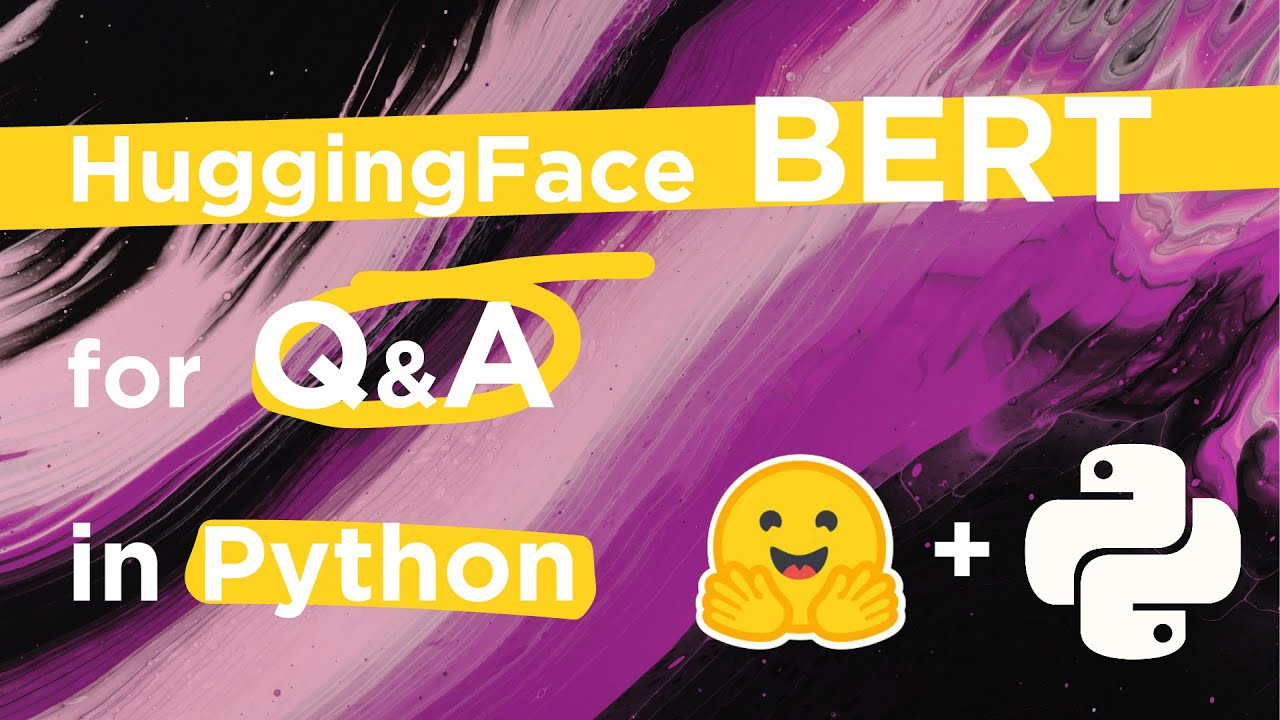# How to Build Q&A Models in Python (Transformers)In this video we'll cover how to build a question-answering model in Python using HuggingFace's Transformers.You will need to install the transformers librar...

https://youtu.be/scJsty_DR3o

Asking a question to a machine and receiving an answer was always the stuff of sci-fi in the not too distant past. Now, things have changed, and we find ourselves using Q&A systems everywhere — without even realizing it.

Google search is the best example — although in most cases Google is used to find information and will simply point you in the right direction, many other queries are direct questions — to which Google often provides direct answers.

Of course, Google is ahead of the game. But that doesn’t mean that we can’t produce great Q&A systems — we can — and we can even use the exact same models that Google uses in their search.

In this video, we will learn how to use state-of-the-art transformer models for Q&A. We will take a look at Google’s Bert and how to use it.

## PyTorch for Deep Learning | Data Science | Machine Learning | Python

PyTorch for Deep Learning | Data Science | Machine Learning | Python. PyTorch is a library in Python which provides tools to build deep learning models. What python does for programming PyTorch does for deep learning. Python is a very flexible language for programming and just like python, the PyTorch library provides flexible tools for deep learning.

## Pytorch vs Tensorflow vs Keras | Deep Learning Tutorial (Tensorflow, Keras & Python)

We will go over what is the difference between pytorch, tensorflow and keras in this video. Pytorch and Tensorflow are two most popular deep learning frameworks. Pytorch is by facebook and Tensorflow is by Google. Keras is not a full fledge deep learning framework, it is just a wrapper around Tensorflow that provides some convenient APIs.

## Handling Imbalanced Dataset in Machine Learning | Deep Learning Tutorial (TensorFlow 2.0 & Python)

In this video I am discussing various techniques to handle imbalanced dataset in machine learning. I also have a python code that demonstrates these different techniques. In the end there is an exercise for you to solve along with a solution link. Credit card fraud detection, cancer prediction, customer churn prediction are some of the examples where you might get an imbalanced dataset. Training a model on imbalanced dataset requires making certain adjustments otherwise the model will not perform as per your expectations.

## PyTorch for Deep Learning | Data Science | Machine Learning | Python

PyTorch is a library in Python which provides tools to build deep learning models. What python does for programming PyTorch does for deep learning.

## PyTorch Tutorial - Deep Learning Using PyTorch - Learn PyTorch from Basics to Advanced

PyTorch Tutorial - Deep Learning Using PyTorch - Learn PyTorch from Basics to Advanced. Learn PyTorch from the very basics to advanced models like Generative Adverserial Networks and Image Captioning. "PyTorch: Zero to GANs" is an online course and series of tutorials on building deep learning models with PyTorch, an open source neural networks library.